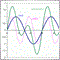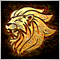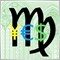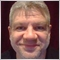# convert iMA function from MT4 to MT555921

Please someone help me with converting iMA function from MT4 to MT5.

I need call iMA with other pair and different shift. I do not see the input shift with the iMA for MT5.
mq5 compiles without error but while testing it give this error:
" (EURJPY,M5)    cannot load indicator 'Moving Average' "

//-----------MT4 code----------------

string symbol = "GBPJPY";
ENUM_TIMEFRAMES TF = 60;           // time frame
int maPeriod = 20;
ENUM_MA_METHOD maMode = MODE_SMA;
ENUM_APPLIED_PRICE priceMethod =  PRICE_CLOSE;
int xShift = 5;       //5 as example or "xShift = i" used with "for(int i=0;i<20;i++)"

double X = iMA(symbol, TF, maPeriod, 0, maMode, priceMethod, xShift ); // this needs to be converted

//-----------MT5 code trial----------------

double X = iMA( symbol, TF,  maPeriod,  xShift ,  maMode,  priceMethod   );   // error

//-----------------------------------

I am not sure if xShift is on the right place with the mq5 function. mq5 provides one parameter less.Moderator
13499

# iMA

The function returns the handle of the Moving Average indicator. It has only one buffer.

int  iMA(
string               symbol,            // symbol name
ENUM_TIMEFRAMES      period,            // period
int                  ma_period,         // averaging period
int                  ma_shift,          // horizontal shift
ENUM_MA_METHOD       ma_method,         // smoothing type
ENUM_APPLIED_PRICE   applied_price      // type of price or handle
);

Parameters

symbol

[in] The symbol name of the security, the data of which should be used to calculate the indicator. The NULL value means the current symbol.

period

[in] The value of the period can be one of the ENUM_TIMEFRAMES values, 0 means the current timeframe.

ma_period

[in] Averaging period for the calculation of the moving average.

ma_shift

[in]  Shift of the indicator relative to the price chart.

ma_method

[in]  Smoothing type. Can be one of the ENUM_MA_METHOD values.

applied_price

[in]  The price used. Can be any of the price constants ENUM_APPLIED_PRICE or a handle of another indicator.

Return Value

Returns the handle of a specified technical indicator,  in case of failure returns INVALID_HANDLE. The computer memory can be freed from an indicator that is no more utilized, using the IndicatorRelease() function, to which the indicator handle is passed.Moderator
13499

//+------------------------------------------------------------------+
//|                                                     Demo_iMA.mq5 |
//|                        Copyright 2011, MetaQuotes Software Corp. |
//|                                              https://www.mql5.com |
//+------------------------------------------------------------------+
#property copyright "Copyright 2011, MetaQuotes Software Corp."
#property link      "https://www.mql5.com"
#property version   "1.00"
#property description "The indicator demonstrates how to obtain data"
#property description "of indicator buffers for the iMA technical indicator."
#property description "A symbol and timeframe used for calculation of the indicator,"
#property description "are set by the symbol and period parameters."
#property description "The method of creation of the handle is set through the 'type' parameter (function type)."
#property description "All other parameters like in the standard Moving Average."

#property indicator_chart_window
#property indicator_buffers 1
#property indicator_plots   1
//--- the iMA plot
#property indicator_label1  "iMA"
#property indicator_type1   DRAW_LINE
#property indicator_color1  clrRed
#property indicator_style1  STYLE_SOLID
#property indicator_width1  1
//+------------------------------------------------------------------+
//| Enumeration of the methods of handle creation                    |
//+------------------------------------------------------------------+
enum Creation
{
Call_iMA,               // use iMA
Call_IndicatorCreate    // use IndicatorCreate
};
//--- input parameters
input Creation             type=Call_iMA;                // type of the function
input int                  ma_period=10;                 // period of ma
input int                  ma_shift=0;                   // shift
input ENUM_MA_METHOD       ma_method=MODE_SMA;           // type of smoothing
input ENUM_APPLIED_PRICE   applied_price=PRICE_CLOSE;    // type of price
input string               symbol=" ";                   // symbol
input ENUM_TIMEFRAMES      period=PERIOD_CURRENT;        // timeframe
//--- indicator buffer
double         iMABuffer[];
//--- variable for storing the handle of the iMA indicator
int    handle;

//--- variable for storing
string name=symbol;
//--- name of the indicator on a chart
string short_name;
//--- we will keep the number of values in the Moving Average indicator
int    bars_calculated=0;
//+------------------------------------------------------------------+
//| Custom indicator initialization function                         |
//+------------------------------------------------------------------+
int OnInit()
{
//--- assignment of array to indicator buffer
SetIndexBuffer(0,iMABuffer,INDICATOR_DATA);
//--- set shift
PlotIndexSetInteger(0,PLOT_SHIFT,ma_shift);
//--- determine the symbol the indicator is drawn for
name=symbol;
//--- delete spaces to the right and to the left
StringTrimRight(name);
StringTrimLeft(name);
//--- if it results in zero length of the 'name' string
if(StringLen(name)==0)
{
//--- take the symbol of the chart the indicator is attached to
name=_Symbol;
}
//--- create handle of the indicator
if(type==Call_iMA)
handle=iMA(name,period,ma_period,ma_shift,ma_method,applied_price);
else
{
//--- fill the structure with parameters of the indicator
MqlParam pars;
//--- period
pars.type=TYPE_INT;
pars.integer_value=ma_period;
//--- shift
pars.type=TYPE_INT;
pars.integer_value=ma_shift;
//--- type of smoothing
pars.type=TYPE_INT;
pars.integer_value=ma_method;
//--- type of price
pars.type=TYPE_INT;
pars.integer_value=applied_price;
handle=IndicatorCreate(name,period,IND_MA,4,pars);
}
//--- if the handle is not created
if(handle==INVALID_HANDLE)
{
//--- tell about the failure and output the error code
PrintFormat("Failed to create handle of the iMA indicator for the symbol %s/%s, error code %d",
name,
EnumToString(period),
GetLastError());
//--- the indicator is stopped early
return(INIT_FAILED);
}
//--- show the symbol/timeframe the Moving Average indicator is calculated for
short_name=StringFormat("iMA(%s/%s, %d, %d, %s, %s)",name,EnumToString(period),
ma_period, ma_shift,EnumToString(ma_method),EnumToString(applied_price));
IndicatorSetString(INDICATOR_SHORTNAME,short_name);
//--- normal initialization of the indicator
return(INIT_SUCCEEDED);
}
//+------------------------------------------------------------------+
//| Custom indicator iteration function                              |
//+------------------------------------------------------------------+
int OnCalculate(const int rates_total,
const int prev_calculated,
const datetime &time[],
const double &open[],
const double &high[],
const double &low[],
const double &close[],
const long &tick_volume[],
const long &volume[],
const int &spread[])
{
//--- number of values copied from the iMA indicator
int values_to_copy;
//--- determine the number of values calculated in the indicator
int calculated=BarsCalculated(handle);
if(calculated<=0)
{
PrintFormat("BarsCalculated() returned %d, error code %d",calculated,GetLastError());
return(0);
}
//--- if it is the first start of calculation of the indicator or if the number of values in the iMA indicator changed
//---or if it is necessary to calculated the indicator for two or more bars (it means something has changed in the price history)
if(prev_calculated==0 || calculated!=bars_calculated || rates_total>prev_calculated+1)
{
//--- if the iMABuffer array is greater than the number of values in the iMA indicator for symbol/period, then we don't copy everything
//--- otherwise, we copy less than the size of indicator buffers
if(calculated>rates_total) values_to_copy=rates_total;
else                       values_to_copy=calculated;
}
else
{
//--- it means that it's not the first time of the indicator calculation, and since the last call of OnCalculate()
//--- for calculation not more than one bar is added
values_to_copy=(rates_total-prev_calculated)+1;
}
//--- fill the iMABuffer array with values of the Moving Average indicator
//--- if FillArrayFromBuffer returns false, it means the information is nor ready yet, quit operation
if(!FillArrayFromBuffer(iMABuffer,ma_shift,handle,values_to_copy)) return(0);
//--- form the message
string comm=StringFormat("%s ==>  Updated value in the indicator %s: %d",
TimeToString(TimeCurrent(),TIME_DATE|TIME_SECONDS),
short_name,
values_to_copy);
//--- display the service message on the chart
Comment(comm);
//--- memorize the number of values in the Moving Average indicator
bars_calculated=calculated;
//--- return the prev_calculated value for the next call
return(rates_total);
}
//+------------------------------------------------------------------+
//| Filling indicator buffers from the MA indicator                  |
//+------------------------------------------------------------------+
bool FillArrayFromBuffer(double &values[],   // indicator buffer of Moving Average values
int shift,          // shift
int ind_handle,     // handle of the iMA indicator
int amount          // number of copied values
)
{
//--- reset error code
ResetLastError();
//--- fill a part of the iMABuffer array with values from the indicator buffer that has 0 index
if(CopyBuffer(ind_handle,0,-shift,amount,values)<0)
{
//--- if the copying fails, tell the error code
PrintFormat("Failed to copy data from the iMA indicator, error code %d",GetLastError());
//--- quit with zero result - it means that the indicator is considered as not calculated
return(false);
}
//--- everything is fine
return(true);
}
//+------------------------------------------------------------------+
//| Indicator deinitialization function                              |
//+------------------------------------------------------------------+
void OnDeinit(const int reason)
{
//--- clear the chart after deleting the indicator
Comment("");
}

It does not return the value like you are used to.

Please see:Documentation on MQL5: Technical Indicators / iMA
• www.mql5.com
Technical Indicators / iMA - Reference on algorithmic/automated trading language for MetaTrader 5[Deleted]
Bernhard Schweigert:

Please someone help me with converting iMA function from MT4 to MT5.

I need call iMA with other pair and different shift. I do not see the input shift with the iMA for MT5.
mq5 compiles without error but while testing it give this error:
" (EURJPY,M5)    cannot load indicator 'Moving Average' "

//-----------MT4 code----------------

string symbol = "GBPJPY";
ENUM_TIMEFRAMES TF = 60;           // time frame
int maPeriod = 20;
ENUM_MA_METHOD maMode = MODE_SMA;
ENUM_APPLIED_PRICE priceMethod =  PRICE_CLOSE;
int xShift = 5;       //5 as example or "xShift = i" used with "for(int i=0;i<20;i++)"

double X = iMA(symbol, TF, maPeriod, 0, maMode, priceMethod, xShift ); // this needs to be converted

//-----------MT5 code trial----------------

double X = iMA( symbol, TF,  maPeriod,  xShift ,  maMode,  priceMethod   );   // error

//-----------------------------------

I am not sure if xShift is on the right place with the mq5 function. mq5 provides one parameter less.

Variable X should be initialized as int, not double.

All else (including function parameters order) looks as correct.

int  iMA(
string               symbol,            // symbol name
ENUM_TIMEFRAMES      period,            // time frame
int                  ma_period,         // MA period
int                  ma_shift,          // MA shift
ENUM_MA_METHOD       ma_method,         // MA method
ENUM_APPLIED_PRICE   applied_price      // price source
);[Deleted]
Bernhard Schweigert:

Please someone help me with converting iMA function from MT4 to MT5.

I need call iMA with other pair and different shift. I do not see the input shift with the iMA for MT5.
mq5 compiles without error but while testing it give this error:
" (EURJPY,M5)    cannot load indicator 'Moving Average' "

//-----------MT4 code----------------

string symbol = "GBPJPY";
ENUM_TIMEFRAMES TF = 60;           // time frame
int maPeriod = 20;
ENUM_MA_METHOD maMode = MODE_SMA;
ENUM_APPLIED_PRICE priceMethod =  PRICE_CLOSE;
int xShift = 5;       //5 as example or "xShift = i" used with "for(int i=0;i<20;i++)"

double X = iMA(symbol, TF, maPeriod, 0, maMode, priceMethod, xShift ); // this needs to be converted

//-----------MT5 code trial----------------

double X = iMA( symbol, TF,  maPeriod,  xShift ,  maMode,  priceMethod   );   // error

//-----------------------------------

I am not sure if xShift is on the right place with the mq5 function. mq5 provides one parameter less.

To avoid error "cannot load indicator" it is better to check if indicator loaded successfully:

if(X == INVALID_HANDLE) Print("Indicator handle loading failed");

Problem with loading indicator could be due to your shift is extending out of rates_total - available bars.55921

Oleg Shenker:

Variable X should be initialized as int, not double.

All else (including function parameters order) looks as correct.

int  iMA(
string               symbol,            // symbol name
ENUM_TIMEFRAMES      period,            // time frame
int                  ma_period,         // MA period
int                  ma_shift,          // MA shift
ENUM_MA_METHOD       ma_method,         // MA method
ENUM_APPLIED_PRICE   applied_price      // price source
);

My intention with varialbe X double was to get the value of the MA, 5 bars back as it was working before with mt4.

I see now the function iMA give back a number of bars calculated and not the value of price. So how can I get the price value of the MA ?

I want to use it as a function and not as a buffer.Moderator
13499

Marco vd Heijden:

It does not return the value like you are used to.

It returns the Handle.

Please see:34992

Bernhard Schweigert:

My intention with varialbe X double was to get the value of the MA, 5 bars back as it was working before with mt4.

I see now the function iMA give back a number of bars calculated and not the value of price. So how can I get the price value of the MA ?

I want to use it as a function and not as a buffer.

You can use my library for porting indicators from MT4 to MT5.55921

Stanislav Korotky:
You can use my library for porting indicators from MT4 to MT5.

Thanks for everybodys help but the more I read about converting mq4 to mq5 the more it becomes complicated.55921

I tried different things but it gets more confusing. Made some changes from your posted stuff. To get things simple but it wasn't.

double getMA(string symbol, ENUM_TIMEFRAMES tf, int MAPeriod, int Shift)
{
int k,end;
end=MAPeriod+Shift;
double X=0;
for(k=Shift;k<end;k++) X+=(iCloseMQL4(symbol,tf,k));
X/=MAPeriod;
Print (symbol," MA = ",X);
return(X);
}

double iCloseMQL4(string symbol,ENUM_TIMEFRAMES tf,int index)
{
double Arr[];
CopyClose(symbol,tf, index, 1, Arr);
Print (symbol," high = ",Arr);
return(Arr);

}

It gave back:

(EURJPY,M5)    EURUSD high = 9.881312916824931e-324

(EURJPY,M5)    EURUSD MA = 9.881312916824931e-32441563

Bernhard Schweigert:

//-----------MT4 code----------------

string symbol = "GBPJPY";
ENUM_TIMEFRAMES TF = 60;           // time frame
int maPeriod = 20;
ENUM_MA_METHOD maMode = MODE_SMA;
ENUM_APPLIED_PRICE priceMethod =  PRICE_CLOSE;
int xShift = 5;       //5 as example or "xShift = i" used with "for(int i=0;i<20;i++)"

double X = iMA(symbol, TF, maPeriod, 0, maMode, priceMethod, xShift ); // this needs to be converted

//-----------MT5 code trial----------------

double X = iMA( symbol, TF,  maPeriod,  xShift ,  maMode,  priceMethod   );   // error

//-----------------------------------

//-----------MT5 code ----------------

// This line should be in OnInit() and maHandle declared as global
int maHandle = iMA( symbol, TF,  maPeriod,  xShift ,  maMode,  priceMethod   );
double
X,mas;

if(CopyBuffer(maHandle,0,xShift,1,mas)!=-1)
X=mas;